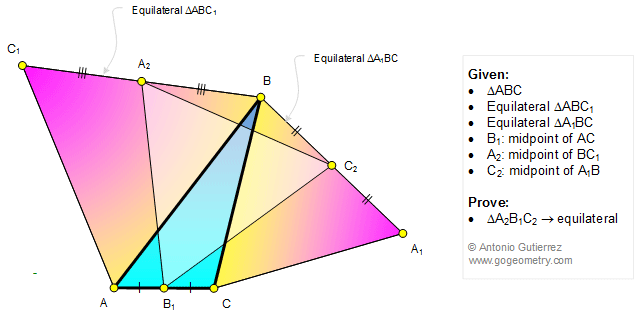# Geometry Problem 1218: Equilateral Triangles, Midpoints, 60 Degrees, Congruence.

< PREVIOUS PROBLEM  |  NEXT PROBLEM >

 In the figure below, equilateral triangles ABC1 and A1BC are drawn on the sides of a triangle ABC. If B1, A2, and C2 are the midpoints of AC, BC1, and A1B, respectively, prove that the triangle A2B1C2 is equilateral.